## 4.1.2 Poisson Equation

The Gauß's law for magnetism (4.4) is satisfied by introduction of the vector potential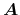as: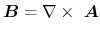(4.5)

Inserting (4.5) into (4.2) yields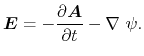(4.6)

Substituting this into the relation of the electric displacement and the electric field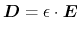(4.7)

results into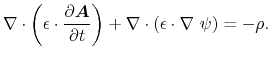(4.8)

The permittivity is considered to be homogenous, therefore the first term in (4.8) is zero due to the definition of(4.5), so the conventional form of the Poisson equation is obtained: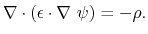(4.9)

The space charge density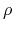can be expressed as the product of the elementary charge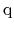and the sum of the electron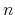and hole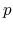concentrations and the net concentration of ionized dopants: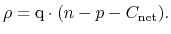(4.10)

Substituting (4.10) into (4.9) gives: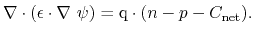(4.11)

S. Vitanov: Simulation of High Electron Mobility Transistors